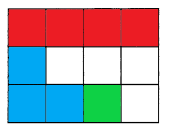Practice the problems of Math in Focus Grade 3 Workbook Answer Key Chapter 14 Fractions to score better marks in the exam.

Challenging Practice

Question 1.
Write a fraction with denominator 9. The fraction should be less than $$\frac{1}{2}$$.
The fraction with denominator 9 and less than 1/2 are 1/9, 2/9, 3/9, 4/9.

Question 2.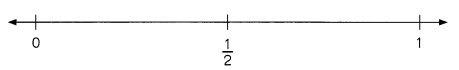Which is greater, $$\frac{3}{7}$$ or $$\frac{2}{3}$$?
__________ > __________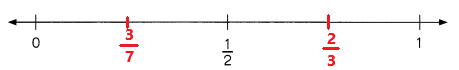2/3 > 3/7
Explanation:
The fraction 2/3 is greater than 3/7.

Question 3.Which is less, $$\frac{1}{8}$$ or $$\frac{2}{5}$$?
__________ < __________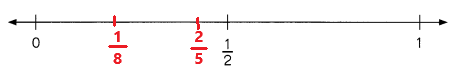1/8 < 2/5
Explanation:
The fraction 1/8 is lees than 2/5.

Question 4.Order $$\frac{1}{7}, \frac{3}{5}, \frac{3}{8}$$, and $$\frac{8}{9}$$ from least to greatest.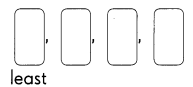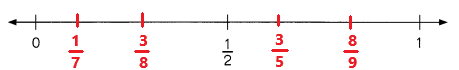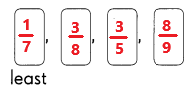Explanation:
The fractions from least to greatest are 1/7, 3/8, 3/5, 8/9.

Problem Solving

Question 1.
Shade to show a fraction greater than $$\frac{1}{4}$$ but less than $$\frac{1}{2}$$.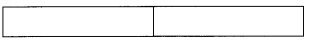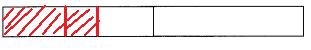Explanation:
In the above image we can observe the shaded part is greater than 1/4 and less than 1/2.
Question 2.
Sam wants to shade $$\frac{2}{3}$$ of the figure. He has already shaded 4 squares. How many more squares must he shade? Help him shade.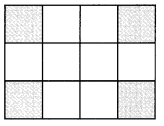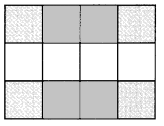Explanation:

Question 3.
Shade the figure to show each fraction below. Use a different color for shading each fraction.
$$\frac{1}{3}, \frac{1}{4}, \frac{1}{12}$$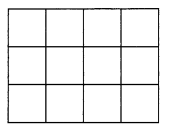What fraction of the figure is unshaded?
___________ of the figure is unshaded.